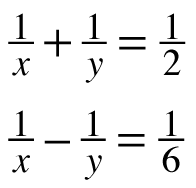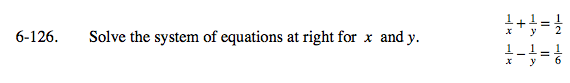### Home > PC > Chapter 6 > Lesson 6.3.2 > Problem6-126

6-126.

Solve the system of equations below for x and y. Homework Help ✎$\frac{2}{x}=\frac{3}{6}+\frac{1}{6}$
$\frac{2}{x}=\frac{4}{6}$